## Hydraulic Press Brake For Sale

We have been producing press brakes for more than 20 years and have rich experience. At the same time, we have established deep relationships with various global technology and parts partners to ensure the stable operation of the press brakes we sell.

### NC Press Brake

If you have limit budget, the NC press brake will be a good choice for you to finish sheet metal bending.

### CNC Press Brake

CNC press brake has much higher accuracy, efficiency than NC press brake when bending metal plate.

### Robotic Press Brake

Robotic press brake It can efficiently and accurately complete the automatic bending of sheet metal.

# How To Calculate Sheet Metal Unfolding?

The first step in the sheet metal process is the unfolding of the geometry and coherent body.

The quality of the part is directly influenced by the correctness and accuracy of the sample unfolding.

In the past, due to the backwardness of calculation tools, people used to use the projection method to enlarge the sample on the plane in 1:1 ratio and measure the real length of the required plain line.

This method is complicated and inefficient, and can no longer meet the needs of social production.

With the development of calculation tools, electronic calculators and the popular application of computers, sheet metal unfolding can be realized by calculation methods.

In order to standardize the calculation method of sheet metal unfolding coefficient of technicians, minimize the dimensional deviation of products after bending, and facilitate the self inspection of workshop personnel and re inspection of quality inspectors, there is a unified standard and basis for the unfolding coefficient, and the calculation standard of sheet metal unfolding coefficient has tended to be standardized.

In the bending process, the outer layer is subjected to tensile stress and the inner layer is subjected to compressive stress.

There is a transition layer between tensile stress and compressive stress – neutral layer.

The length of the neutral layer during bending is the same as that before bending, so the neutral layer is the benchmark for calculating the unfolded length of the bending part.

The position of neutral layer is related to the degree of deformation.

When the bending radius is large and the bending angle is small, the deformation degree is small, and the position of the neutral layer is close to the center of the sheet thickness;

When the bending radius (R) becomes smaller and the bending angle (θ) increases, the degree of deformation increases and the position of the neutral layer gradually moves to the inner side of the bending center.

The distance from the neutral layer to the inner side of the sheet is denoted by λ, and the material thickness is denoted by T.

The basic formula for calculating the unfold length:

Unfolding length = inner size of material + inner size of material + amount of compensation.

The following describes the unfolding algorithms of various bend features one by one.

## General bending I

R = 0mm, θ = 90° (Note: When R ≤ 1.0mm, it is treated as R = 0mm).

L = A + B + K

• When 0 < T ≤ 3mm, K = 0
• When 0.3mm < T ≤ 5T, K = 0.4T

In actual production, usually bending upper die wear with R angle, or would have less than 1mm R angle, or the choice of the lower die V slot is large and other reasons, resulting in a small bending coefficient.

Therefore, according to the actual empirical value, the bending coefficient of each material plate thickness is shown in Table 1.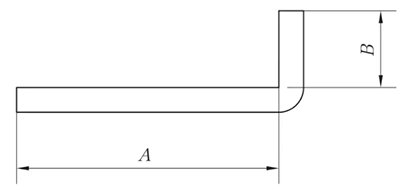Fig. 1 Schematic diagram of general bending I

## General bending II

R ≠ 0mm and θ = 90°

• When 0 < T ≤ 6mm, λ = 0.5T
• When 0 < T ≤ 6mm, λ = 0.5T
• When T > 1.6mm, λ = 0.4T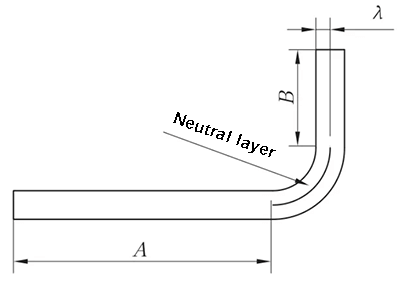Fig. 2 Schematic diagram of general bending II

## General bending III

R = 0mm, θ ≠ 90°

L = A + B + K’

• When T ≤ 3mm, K’ = 0
• When T > 0.3mm, K’ = (θ/90° )×K

Note: K is the compensation amount at 90°.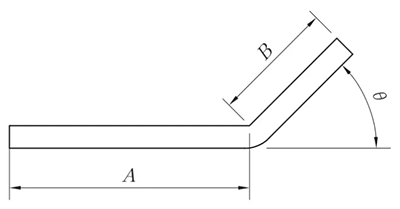## General bending IV

R ≠ 0mm, θ ≠ 90°

L = A + B + K

(K takes the neutral layer arc length)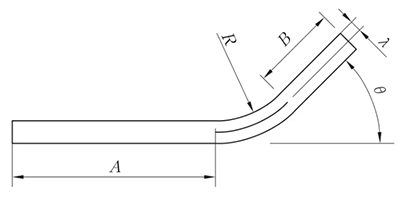Fig. 4 General bending IV bending diagram

The unfolding method is the same as the parallel straight-edge Z-fold method, and the height is taken as shown in Figure 6.

The angle θ can be regarded as 90° unfolding.

When T > 1.6 mm, λ = 0.4T.

## Z-bend

Z-fold, also known as offset bending, is divided into straight edge offset bending and bevel edge offset bending according to the forming angle, and the processing method depends on the offset height.

• When the offset height h is less than 3.5 times the material thickness, the offset die or changeable die is used for forming.
• When the offset height is more than 3.5 times the material thickness, the normal positive and negative forming is used.
• When the length of the beveled edge is less than 3.5 times the material thickness, use the offset die or changeable die forming.
• When the length of beveled edge is more than 3.5 times the material thickness, the normal one positive and one negative folding is used.

### Z-fold 1 (straight edge offset)

(1) When H < 3.5T, only then can be processed by offset bending.

L = A + B + H

(if H ≤ T, then compensate 0.2mm)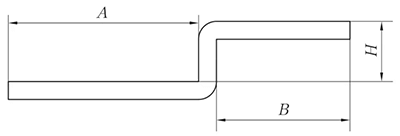Fig. 5 Schematic diagram of straight edge offset

(2) Non parallel straight edge offset

L = A + B + K

(K is the arc length of neutral layer)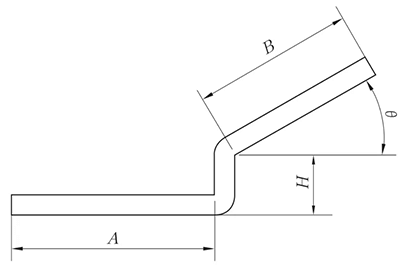Fig. 6 Schematic diagram of non parallel straight edge offset

(3) Straight edge offset – the transition segment is tangent to two arcs

When the straight edge offset unfolded at the tangent point of two circular arcs, a vertical line is made at the tangent point of two circular arcs on the outside of the substrate, and then the material is offset one material thickness to the inside, as shown in Figure 7, for processing, and unfolded in the way of Z-fold 1 (straight edge offset).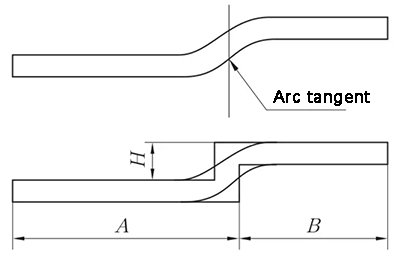Fig. 7 Straight edge offset diagram – transition segment is tangent to two circular arcs

### Z-fold 2 (bevel offset)

When H < 2T, the unfolding algorithm is as follows according to the offset processing.

• When θ ≤ 70°, L = A + B + C + 0.2
• When θ > 70°, unfold according to Z-fold 1 (straight edge offset)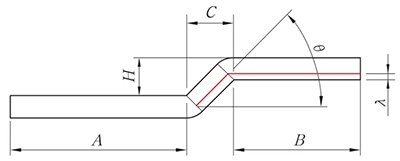Fig. 8 Diagram of beveled edge offset

## Reverse folding and flattening

L = A + B – K

(1) When flattening, consider whether to press the line before bending according to the actual situation. The line pressing position is in the middle of the bending deformation area.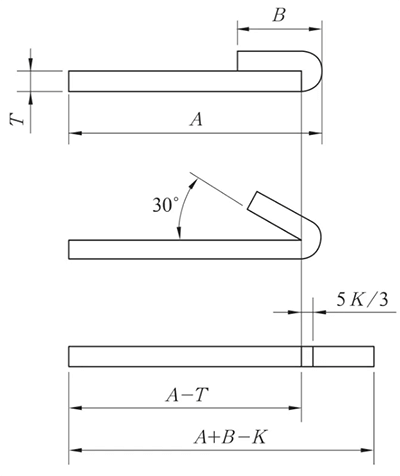Fig. 9 Schematic diagram of reverse folding and flattening

The reverse folding and flattening is generally carried out in two steps: first bend to 30 ° with the insertion die, and then flatten.

Therefore, when making the bending line of the expanded drawing, it is necessary to draw the bending line according to the 150 ° bending, as shown in Figure 9.

Table 1 bending coefficient of plates with different thickness under different bending angles

 Angle Thickness/mm 1 1.2 1.5 1.8 2.5 45° 5.3 6.3 7.8 9.5 13 50° 4.5 5.4 6.8 8.1 11.25 55° 4 4.7 5.8 7.05 9.75 60° 3.4 4.1 5.1 6.15 8.5 65° 3 3.6 4.5 5.4 7.5 70° 2.65 3.2 4 4.75 6.6 75° 2.35 2.8 3.5 4.25 5.9 80° 2.1 2.5 3.1 3.75 5.25 85° 1.9 2.25 2.8 3.35 4.65 90° 1.7 2 2.5 3 4.15 95° 1.5 1.8 2.2 2.7 3.75 100° 1.35 1.6 2 2.4 3.35 105° 1.2 1.4 1.75 2.15 3 110° 1.1 1.3 1.6 2 2.65 115° 1 1.25 1.4 1.7 2.35 120° 0.85 1 1.25 1.5 2.1 125° 0.75 0.9 1.1 1.35 1.85 130° 0.65 0.8 1 1.18 1.65 135° 0.55 0.7 0.85 1.05 1.45 140° 0.5 0.6 0.75 0.9 1.25 145° 0.43 0.5 0.65 0.77 1.05 150° 0.35 0.43 0.55 0.65 0.9 155° 0.3 0.35 0.45 0.53 0.75 160° 0.23 0.27 0.35 0.4 0.6 165° 0.17 0.2 0.25 0.3 0.45

## N–fold

The N-fold processing method is shim refolding and flattening, and the unfolding algorithm is:

L = A + B + K

(K takes the neutral layer arc length).

λ = 0.5T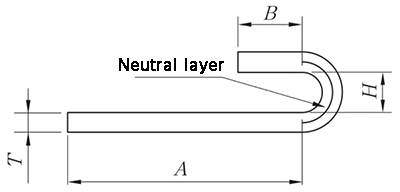Fig.10 N-fold diagram

## Arc (including straight edge on one side)

(1) The arc expansion is directly K, and the arc length of the neutral layer is taken. λ＝ 0.5T

(2) Expansion with straight edge on one side.

L = A + K

(k is the arc length of neutral layer)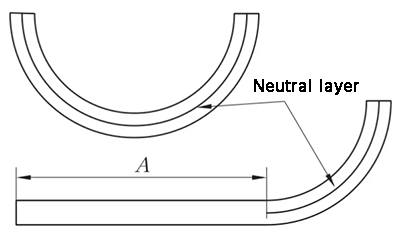Fig. 11 Schematic diagram of arc bending

## Conclusion

Sheet metal parts are widely used in electromechanical, light industry, automobile industries.

The unfolded shape of sheet metal parts is the main basis of blanking size, which determines the size and shape of blanking.

In the past, the traditional unfolding methods of sheet metal parts have become more and more unsuitable to the modern design requirements due to the disadvantages of long cycle, low efficiency and poor quality.

In order to overcome these disadvantages, some advanced CAD systems are gradually increasing in recent years. These special sheet metal parts design systems have powerful functions and save a lot of time and manpower for the preliminary design and parts deployment of sheet metal.